# Synchronization behavior of a weakly damped far-resonance vibrating system

## Bang Chen1, Xiao’ou Xia2, Xiaobo Wang3

1, 2School of Mechanical Engineering, University of Science and Technology Beijing, Beijing, China

3BGRIMM Technology Group, Beijing, China

1Corresponding author

Vibroengineering PROCEDIA, Vol. 20, 2018, p. 134-139. https://doi.org/10.21595/vp.2018.20199
Received 7 September 2018; accepted 17 September 2018; published 19 October 2018

34th International Conference on Vibroengineering in Shanghai, China, October 19-21st, 2018

Copyright © 2018 Bang Chen, et al. This is an open access article distributed under the Creative Commons Attribution License, which permits unrestricted use, distribution, and reproduction in any medium, provided the original work is properly cited.
Views 45
CrossRef Citations 0
Abstract.

In order to reveal synchronization characteristics of a weakly damped system with two rotors mounted on different vibrating bodies, we propose a simplified physical model. Vibration of the system is discussed by the average method, which can separate fast motions (high frequency) from slow motions (low frequency). Theoretical research shows that vibration torque is the key factor to balance the energy distribution between rotors. For the system with rotational frequencies larger than the nature frequencies, the coupling characteristic frequency or characteristic frequency curve should be considered. As the coupling frequency is close to the characteristic frequency, or the vibration state is close to the characteristic frequency curve, self-synchronization of two rotors can be obtained easily.

Keywords: rotor, exciter, self-synchronization, simulation, vibration synchronization.

#### 1. Introduction

The so-called self-synchronization phenomenon corresponds to the consistency or certain relationship of systems’ parameters caused by their internal couplings and has been widely involved in non-linear vibration, hydraulic [1-3], electromechanical coupling, automatic control theory and other fields [4-8].

Huygens was the first person who observed the synchronization of pendulum clocks in the 17th Century. In [4, 5], Czolczynski et al. presented different synchronous behaves of two or $n$ pendula installed on a frame. The self-synchronization theory of rotors was developed by Bleckman [1, 2] with averaging method in the middle of the 20th century. Wen and Zhao et al.  modified the averaging method and proposed the average method with two small parameters. Zhang  deduced the synchronization condition and the synchronization stability for the vibrating system with three rotors. Hou and Fang [9, 10] investigated a vibrating screen based on the model of a rotor-pendulum system.

The above researches are mostly focused on the synchronization of pendula or rotors installed on the same vibrating frame. In this paper, we propose a vibrating system with two rotors mounted on two different vibrating bodies.

#### 2. Dynamical equations of the vibrating system

As shown in Fig. 1, two rotors are mounted on different vibrating bodies. The vibrating body (${M}_{i}$) ($i=$ 1, 2) can move in horizontal direction (${x}_{i}$) and is installed on the foundation by the spring. The two bodies are connected by a coupling spring. Counterclockwise direction is taken as positive. Inertia moment and eccentricity of the rotor on its mass center are given by ${j}_{i}$ and ${r}_{i}$. Other variables are show in Fig. 1. In this paper, synchronization of rotors is analyzed in a non-resonant vibrating system, in which rotation frequencies of rotors are larger than nature frequencies of vibrating bodies. The system is denoted as after-resonance system. We assume that ${\stackrel{˙}{\phi }}_{1}$, ${\stackrel{˙}{\phi }}_{2}>\text{2}{\omega }_{1}$, $\text{2}{\omega }_{2}$, where ${\omega }_{1}=\sqrt{{k}_{1}/{M}_{1}}$, ${\omega }_{2}=\sqrt{{k}_{2}/{M}_{2}}$.

Fig. 1. Simplified model of the system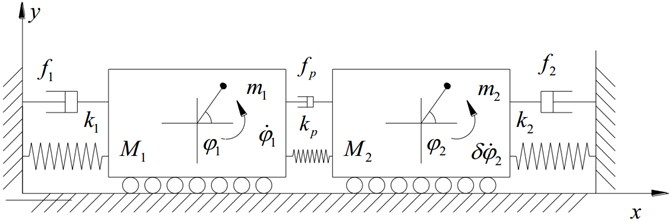The electromagnetic torque and resistance moment of the driving motor are ${T}_{ei}$ and ${T}_{fi}$ (${T}_{fi}={f}_{ri}\bullet {\stackrel{˙}{\phi }}_{i}$) respectively. When ${\stackrel{˙}{\phi }}_{i}$ ($i=$ 1, 2) fluctuates near the frequency ${\omega }_{n}$, the influence of electromagnetic leakage can be neglected, and the driving force of induction motor can be linearized as ${T}_{ei}=n{L}_{mi}^{2}{U}_{0}^{2}\left({\omega }_{si}-n{\omega }_{n}\right)/\left({{L}_{si}^{2}{\omega }_{si}{R}_{ri}\omega }_{si}\right)$, where ${n}_{i}$, ${L}_{mi}$, ${\omega }_{si}$, ${L}_{si}$ and ${R}_{ri}$ ($i=$1, 2) correspond to the pole number, mutual inductance, synchronous speed, stator inductance and rotor resistance of the motor; ${U}_{0}$ is the voltage amplitude. As self-synchronization of rotors is achieved, speed fluctuations of rotors are small [1, 2]. Therefore, small variables can be neglected. Introducing ${\omega }_{p}=\sqrt{{k}_{p}/{M}_{1}}$, $\eta ={M}_{2}/{M}_{1}$, ${J}_{1}={j}_{1}+{m}_{1}{r}_{1}^{2}$ and ${J}_{2}={j}_{2}+{m}_{2}{r}_{2}^{2}$, we have:

(1)
${\stackrel{¨}{x}}_{1}+{\omega }_{1}^{2}{x}_{1}+\eta {\omega }_{p}^{2}\left({x}_{1}-{x}_{2}\right)=\frac{{m}_{1}}{{M}_{1}}{r}_{1}{\stackrel{˙}{\phi }}_{1}^{2}\mathrm{cos}{\phi }_{1},$
${\stackrel{¨}{x}}_{2}+{\omega }_{2}^{2}{x}_{2}+\eta {\omega }_{p}^{2}\left({x}_{2}-{x}_{1}\right)=\frac{{m}_{2}}{{M}_{2}}\delta {r}_{2}{\stackrel{˙}{\phi }}_{2}^{2}\mathrm{cos}{\phi }_{2},$
${J}_{1}{\stackrel{¨}{\phi }}_{1}={T}_{e1}-{T}_{f1}+{m}_{1}{r}_{1}{\stackrel{¨}{x}}_{1}\mathrm{sin}{\phi }_{1},$
${J}_{2}{\stackrel{¨}{\phi }}_{2}={T}_{e2}-{T}_{f2}+\delta {m}_{2}{r}_{2}{\stackrel{¨}{x}}_{2}\mathrm{sin}{\phi }_{2}.$

The synchronous speed of two rotors is denoted by ${\omega }_{n}$. When self-synchronization of rotors is achieved [1, 3], phases of the two rotors can be denoted as ${\phi }_{1}={\omega }_{n}t+{\alpha }_{1}$, ${\phi }_{2}={\omega }_{n}t+{\alpha }_{2}$, where ${\alpha }_{1}$ and ${\alpha }_{2}$ are slowly-varying parameters. From Eq. (1), we obtain:

(2)
${x}_{1}={\mu }_{11}\mathrm{cos}{\phi }_{1}+{\mu }_{12}\mathrm{cos}{\phi }_{2},$
${x}_{2}={\mu }_{21}\mathrm{cos}{\phi }_{1}+{\mu }_{22}\mathrm{cos}{\phi }_{2},$

where:

$N={M}_{1}\left[\left({\omega }_{1}^{2}+\eta {\omega }_{p}^{2}-{\omega }_{n}^{2}\right)\left({\omega }_{2}^{2}+{\omega }_{p}^{2}-{\omega }_{n}^{2}\right)-\eta {\omega }_{p}^{4}\right].$

${\mu }_{11}$, ${\mu }_{12}$, ${\mu }_{21}$ and ${\mu }_{22}$ show the coupling effects in the system. Rotors are driven by motors, and the resistance is approximately proportional to its speed. The average values of resultant torques of rotors are denoted by ${P}_{1}$, ${P}_{2}$. As the system is stable, we have:

(3)
${P}_{1}={\int }_{t}^{t+T}{T}_{e1}-{T}_{f1}+{m}_{1}{r}_{1}{\stackrel{¨}{x}}_{1}\mathrm{sin}{\phi }_{1}dt={T}_{e1}\left({\omega }_{n}\right)-{T}_{f1}\left({\omega }_{n}\right)-\frac{1}{2}{T}_{v},$
${P}_{2}={\int }_{t}^{t+T}{T}_{e2}-{T}_{f2}+\delta {m}_{2}{r}_{2}{\stackrel{¨}{x}}_{2}\mathrm{sin}{\phi }_{2}dt={T}_{e2}\left({\omega }_{n}\right)-{T}_{f2}\left({\omega }_{n}\right)+\frac{1}{2}{T}_{v},$

where ${T}_{v}=\delta {m}_{2}{r}_{2}{\mu }_{21}{\omega }_{n}^{2}\mathrm{sin}\left({\alpha }_{1}-{\alpha }_{2}\right)={m}_{1}{r}_{1}{\mu }_{12}{\omega }_{n}^{2}\mathrm{sin}\left({\alpha }_{1}-{\alpha }_{2}\right)$ is the vibration torque (VT). Introducing the variable substitutions:

$\mathrm{sin}∆\alpha =\frac{\left[∆{T}_{e}\left({\omega }_{n}\right)-∆{T}_{f}\left({\omega }_{n}\right)\right]}{\left({m}_{1}{r}_{1}{\mu }_{12}{\omega }_{n}^{2}\right)},$

can be obtained. Thus, the synchronization condition can be expressed as follows :

(4)
$\left|\left[∆{T}_{e}\left({\omega }_{n}\right)-∆{T}_{f}\left({\omega }_{n}\right)\right]\bullet \frac{1}{\mathrm{\delta }{m}_{2}{r}_{2}{\mu }_{21}{\omega }_{n}^{2}}\right|\le 1.$

The stability criterion of the synchronous state can be discussed based on Lyapunov stability theory, it can be deduced as:

(5)
$\frac{\delta }{\left({\omega }_{1}^{2}+\eta {\omega }_{p}^{2}-{\omega }_{n}^{2}\right)\left({\omega }_{2}^{2}+{\omega }_{p}^{2}-{\omega }_{n}^{2}\right)-\eta {\omega }_{p}^{4}}\mathrm{cos}∆\alpha >0.$

#### 3. Discussions of theoretical results

In this paper, two rotors rotate in the same direction, that is, $\delta =$ 1. In this paper, ${L}_{mi}=$ 0.14 H, ${L}_{si}=$ 0.12 H, ${R}_{ri}=$ 0.6 $\mathrm{\Omega }$, ${\omega }_{si}=$ 314 rad/s, ${U}_{0}=$ 220 V, $n=$ 2 and other parameters of the system are shown in Table 1.

Table 1. Parameters of the system

 Parameters ${M}_{i}$ [kg] ${m}_{i}$ [kg] ${j}_{i}$ [kg·m2] ${r}_{i}$ [m] ${f}_{i}$ [N·s/m] ${k}_{i}$ [N/m] ${f}_{ri}$ [N·m·s/rad] Rotor 1 300 3.5 0.3 0.15 200 7.5×105 3×10-2 Rotor 2 200 2.5 0.3 0.1 200 7.4×105 1.47×10-1

We take ${T}_{v\mathrm{m}\mathrm{a}\mathrm{x}}\left({\omega }_{p}^{2}\right)=\delta {m}_{1}{r}_{1}{m}_{2}{r}_{2}{\omega }_{n}^{4}{\omega }_{p}^{2}/N$ into consideration. ${T}_{v}=\mathrm{sin}∆\alpha {T}_{v\mathrm{m}\mathrm{a}\mathrm{x}}\left({\omega }_{p}^{2}\right)$ can be obtained easily. Thus, ${T}_{v\mathrm{m}\mathrm{a}\mathrm{x}}\left({\omega }_{p}^{2}\right)$ is the maximum vibration torque of the system (MVT). For the after-resonance system, denominator of $N$ may be zero when ${\omega }_{p}$ come to be a specific value $\chi$. ${\chi }^{2}$ is deduced as:

(6)
$\chi =\sqrt{\frac{\left({\omega }_{1}^{2}-{\omega }_{n}^{2}\right)\left({\omega }_{2}^{2}-{\omega }_{n}^{2}\right)}{{\omega }_{n}^{2}-{\omega }_{1}^{2}+\eta \left({\omega }_{n}^{2}{-\omega }_{2}^{2}\right)}}.$

$\chi$ is called the characteristic frequency (CF) of the system, as shown in Fig. 2(a) and Fig. 3. In the coordinates of ${\omega }_{n}$ and ${\omega }_{p}^{2}$, the characteristic curve composed of characteristic frequencies at different synchronous speeds is shown in Fig. 2(b). The curve of ${T}_{v\mathrm{m}\mathrm{a}\mathrm{x}}\left({\omega }_{p}^{2}\right)$ accompanied with ${\omega }_{p}^{2}$ is shown in Fig. 3 when ${\omega }_{n}$ is a certain value (for example, ${\omega }_{n}=$ 155 rad/s). The synchronous speed varies with the stiffness of the coupling spring and it can be obtained by numerical simulation.

When ${\omega }_{p}^{2}$ approach ${\chi }^{2}$ from the left side, ${T}_{v\mathrm{m}\mathrm{a}\mathrm{x}}$ tends to infinity; when ${\omega }_{p}^{2}$ continues to increase over ${\chi }^{2}$ to infinity, ${T}_{v\mathrm{m}\mathrm{a}\mathrm{x}}$ gradually decreases and tends to a constant value. In Fig. 3, the curve ${T}_{v\mathrm{m}\mathrm{a}\mathrm{x}}$ is divided into four parts by the curves of $±\left(∆{T}_{e}\left({\omega }_{n}\right)-∆{T}_{f}\left({\omega }_{n}\right)\right)$. The four parts are denoted by LA (passing through point A), LB (passing through point B), LC (passing through point C) and LD (passing through point D) respectively. According to Eq. (4), self-synchronization of two rotors cannot be obtained when system state occurs on LA and LD; On the contrary, rotations of two rotors can be self-synchronizing when system state occurs on LB and LC.

As coupling frequency ${\omega }_{p}$ is close to the characteristic frequency $\chi$, or the system state is near the characteristic frequency curve, the system coupling performance is strong, and the self synchronization of two rotors can be obtained easily. And it is convenient to control the synchronization performance by adjusting the coupling spring stiffness ${k}_{p}$.

Fig. 2. Surface of the maximum vibration momentvarying with the coupling frequency and synchronous speed in the after-resonance system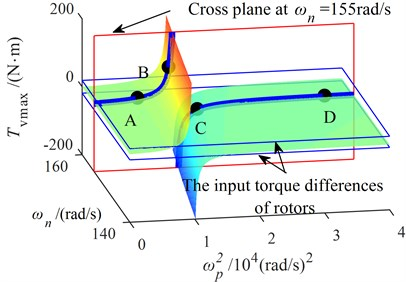a) The three-dimensional diagram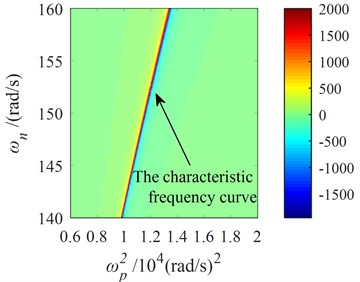b) The two-dimensional diagram

Fig. 3. Relationship between the maximum vibration moment and coupling frequency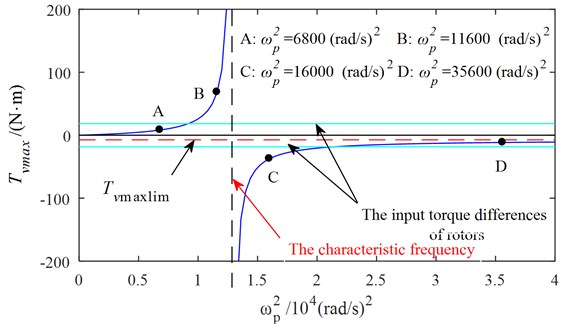#### 4. Simulations for synchronization of two rotors

Simulations are carried out with ${\omega }_{p}^{2}$ set to be 6800 (rad/s)2, 11600 (rad/s)2, 16000 (rad/s)2, 35600 (rad/s)2 and infinity respectively, corresponding to points A, B, C and D.

Fig. 4 shows the simulation results with ${\omega }_{p}^{2}=$ 6800 (rad/s)2. From Figs. 2 and 3, we can suggest that rotations of two rotors would not be self-synchronizing in this case. As shown in Fig. 4, speeds of the two rotors are not consistent. Fig. 5 shows the simulation results with ${\omega }_{p}^{2}=$ 11600 (rad/s)2. In this case, $\left|{T}_{v\mathrm{m}\mathrm{a}\mathrm{x}}\right|>\left|{∆T}_{e}\left({\omega }_{n}\right)-{∆T}_{f}\left({\omega }_{n}\right)\right|$. $∆\alpha$ is calculated to be 3.28 rad. As shown in Fig. 5, speeds of the two rotors reach the same value around 1.5 s, and $∆\alpha$ is stable at 15.83 rad (15.83 – 4 × = 3.26 (rad)). Fig. 6 shows the simulation results with ${\omega }_{p}^{2}=$ 16000 (rad/s)2. Parameters of system satisfy the self-synchronization conditions in this case too. $∆\alpha$ is calculated to be 3.65 rad. As shown in Fig. 6, speeds of the two rotors reach the same value around 1.5 s, and $∆\alpha$ is stable at 16.21 rad (16.21 – 4 × $\pi$ = 3.64 (rad)). The numerical results in Figs. 5 and 6 are consistent with the theoretical analysis.

Fig. 4. Simulation results of the after-resonance system when the coupling stiffness is 1.36×106 N/m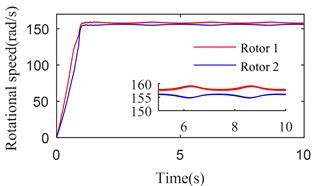a) Rotational speeds of two rotors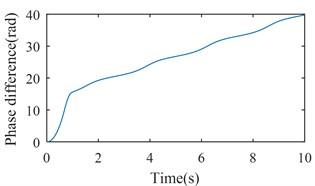b) The phase difference between two rotors

Fig. 5. Simulation results of the after-resonance system when the coupling stiffness is 2.32×106 N/m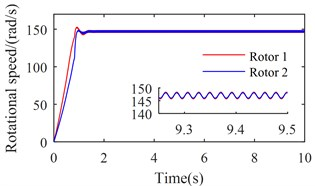a) Rotational speeds of two rotors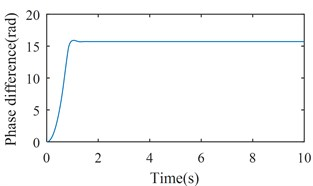b) The phase difference between two rotors

Fig. 6. Simulation results of the after-resonance system when the coupling stiffness is 3.20×106 N/m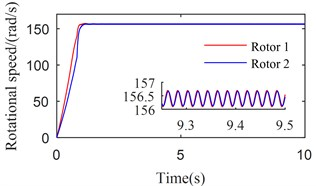a) Rotational speeds of two rotors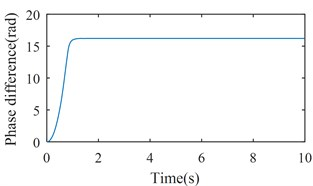b) The phase difference between two rotors

Fig. 7. Simulation results of the after-resonance system when the coupling stiffness is 7.12×106 N/m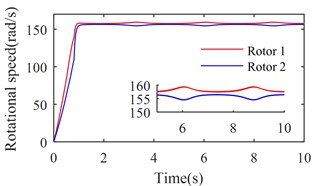a) Rotational speeds of two rotors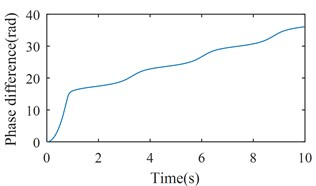b) The phase difference between two rotors

Fig. 8. Simulation results of the after-resonance system when the coupling stiffness is tending to infinity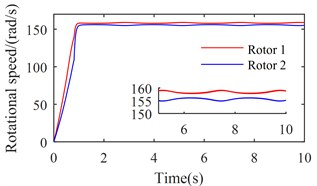a) Rotational speeds of two rotors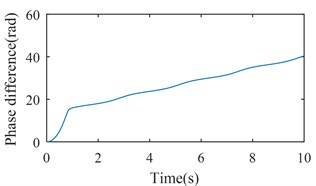b) The phase difference between two rotors

Figs. 7 and 8 show the simulation results when ${\omega }_{p}^{2}$ is set to be 35600 (rad/s)2 and tend to infinity respectively. According to Figs. 2 and 3, rotations of two rotors would not be self-synchronizing in these cases. As shown in Figs. 7(a) and 8(a), speeds of the two rotors are not consistent when the system reaches stable conditions; Figs. 7(b) and 8(b) demonstrate the asynchrony of the two rotors. Simulation results confirm the theoretical analysis.

#### 5. Conclusions

Self-synchronization of two rotors can be observed in a weakly damped non-resonant vibrating system with two rotors mounted on different bodies. The synchronization condition is that the vibration torque is large enough to overcome the input torque difference between two rotors. For the after-resonance system, there is a characteristic frequency or characteristic frequency curve. As the coupling frequency is close to the characteristic frequency, the coupling effects of the system can be strong, and self-synchronization of two rotors occurs easily. While there is a big difference between the coupling frequency and the characteristic frequency, self-synchronization will not be achieved.

#### Acknowledgements

This study is supported by International Science and Technology Cooperation Program of China (Grant No. 2015DFR70660).

1. Blekhman I. I. Synchronization in Science and Technology. ASME Press, New York, USA, 1988. [Search CrossRef]
2. Blekhman I. I. Vibrational Mechanics. World Scientific, Singapore, 2000. [Publisher]
3. Wen B. C., Fan J., Zhao C. Y., Xiong W. L. Vibratory Synchronization and Controlled Synchronization in Engineering. Science Press, Beijing, China, 2009. [Search CrossRef]
4. Czolczynski K., Perlikowski P., Stefanski A., Kapitaniak T. Clustering of Huygens’ clocks. Progress of Theoretical Physics, Vol. 122, Issue 4, 2009, p. 1027-1033. [Publisher]
5. Czolczynski K., Perlikowski P., Stefanski A., Kapitaniak T. Why two clocks synchronize: energy balance of the synchronized clocks. Chaos, Vol. 21, Issue 2, 2011, p. 023129. [Publisher]
6. Wen B. C., Zhang H., Liu S. Y., He Q., Zhao C. Y. Theory and Techniques of Vibrating Machinery and Their Applications. Science Press, Beijing, China, 2010. [Search CrossRef]
7. Zhao C. Y., Zhu H. T., Zhang Y. M. Synchronization of two coupled exciters in a vibrating system of spatial motion. Acta Mechanica Sinica, Vol. 26, Issue 3, 2010, p. 477-493. [Publisher]
8. Zhang X. L., Wen B. C., Zhao C. Y. Experimental investigation on synchronization of three co-rotating non-identical coupled exciters driven by three motors. Journal of Sound and Vibration, Vol. 333, Issue 13, 2014, p. 2898-2908. [Publisher]
9. Fang P., Hou Y. J., Nan Y. H., Yu L. Study of synchronization for a rotor-pendulum system with Poincare method. Journal of Vibroengineering, Vol. 17, Issue 5, 2015, p. 2681-2695. [Search CrossRef]
10. Fang P., Yang Q. M., Hou Y. J., Chen Y. Theoretical Study on self-synchronization of two homodromy rotors coupled with a pendulum rod in a far-resonant vibrating system. Journal of Vibroengineering, Vol. 16, Issue 5, 2014, p. 2188-2695. [Search CrossRef]